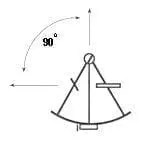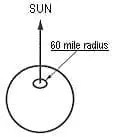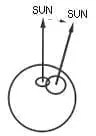# How Does Celestial Navigation Work

1. Imagine that the sun is directly overhead at a particular time. Then we simply look into the Nautical Almanac to find out where the sun is; and that is where we are!2. How do we know the sun is directly overhead? The sextant tells us. It measures the angle from the horizon (meaning a level point) up to the sun. In this case the angle would be 90°.
3. But what if the sun were 1° away from overhead (sextant reads 89°)? Then we would be somewhere on a circle 60 miles (60 miles = 1°) from where the Nautical Almanac said the sun was. This is a circular line of position (LOP).4. An actual position occurs where two lines of position (LOPs) cross. For example: where Interstate 80 and Interstate 35 cross; or where your latitude and longitude cross, or where the above circular LOP crosses another LOP.
5. If we look at the sun later in the day, we would get a second circle, and our position would be at one of the intersections (if you don’t know which one, do a third circle to settle the matter).### 2 thoughts on “How Does Celestial Navigation Work”

1.Bob Doran says:

How can a “sun line’ be used to find correct latitude ? IE: Sunrise at Long 176 deg-44 min, 0 deg- very near the Equator

•Amy Gebhart says:

Sun lines at sunrise give only longitude.